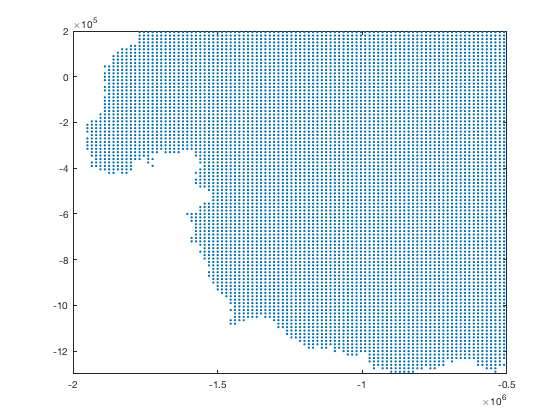The xyzread function simply imports the x,y,z columns of a .xyz file. Note: there is no real standard for .xyz files, so your .xyz file may be different from the .xyz files I wrote this for. I wrote this one for GMT/GIS files. See also xyz2grid.

Back to Climate Data Tools Contents

## Description

[x,y,z] = xyzread(filename) imports the columns of a plain .xyz file.

[x,y,z] = xyzread(filename,Name,Value) accepts any textscan arguments such as 'headerlines' etc.

## Example

For this example, use load Antarctic Curie depth data from Martos 2017:

```[x,y,z] = xyzread('Curie_Depth.xyz');
```

The syntax above is equivalent to

```[x,y,z] = xyzread('Curie_Depth.xyz','headerlines',0);
```

because this particular xyz file does not have any header lines.

We have now loaded the data into Matlab and it looks like this:

```whos
```
```  Name          Size             Bytes  Class     Attributes

x         59328x1             474624  double
y         59328x1             474624  double
z         59328x1             474624  double

```

We have three variables, each 59328x1 in size. Here are the x,y coordinates plotted as dots:

```plot(x,y,'.')
```At this zoom level, that looks like a solid continent, but only because the ~60,000 dots are all squished together. Zoom in closer to see the regularity of the grid:

```axis([-2 -0.5 -1.3 .2]*1e6)
```Those dots are regularly spaced in x and y, but getting them into a regular grid in Matlab is kind of a noodle scratcher. Fortunately, xyz2grid makes it easy!

```[X,Y,Z] = xyz2grid(x,y,z);

whos X Y Z
```
```  Name        Size              Bytes  Class     Attributes

X         291x350            814800  double
Y         291x350            814800  double
Z         291x350            814800  double

```

Now those 59328x1 arrays have been neatly packed into 291x350 grids which can be plotted with pcolor:

```pcolor(X,Y,Z)